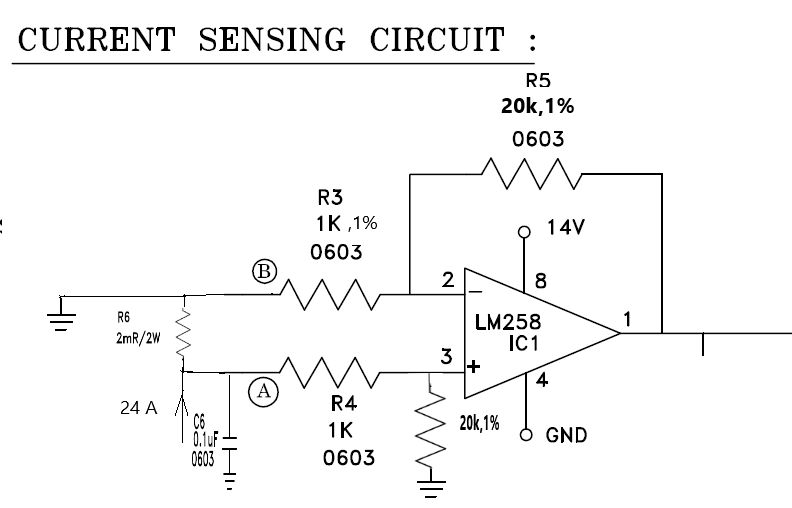If you have a related question, please click the "Ask a related question" button in the top right corner. The newly created question will be automatically linked to this question.

# LM258A: Opamp Gain Error

Part Number: LM258A

I m using lm258A as differential amplifier for current sensing. Op-amp gain is more than calculated value .

For 24 A, 2 mohm ,

Vd=0.048,Gain = 20

Vout = 0.048*20=0.96V .

But i m getting Vout as 3V @ 24 A in practical case.

All the resistors are 1 % tolerance .Please find the attached circuit

.Please give solution to solve the more op-amp gain• Part Number: LM258A

I m using opamp as differential amplifier for current sensing .Opamp gain is more than calculated valueThe resistors used in the circuit are 1% tolerance .

I m using 2mohm,24A

Vd =0.048 v ,Gain = 20

Vout = 0.96V but I got Vout = 3V .

Huge gain variation found in testing.

Pls give solution for this opamp Gain error.• Jayashree,

There many factors that create small differences. Due to the large error and very small sense resistor, I suggest looking at the kelvin connection on the sense resistor very carefully.

If that looks OK, test results at 0A, 8A, 16A too.

• Hi Jayashree,

keep in mind that a solder joint can already have a resistance of 1mR. So it plays an enormeous role where exactly you "touch" the shunt.

Also, I would use +/-0.1% toleranced resistors in the differential amplifier. A better resistor match results in a much better common mode rejection :-)